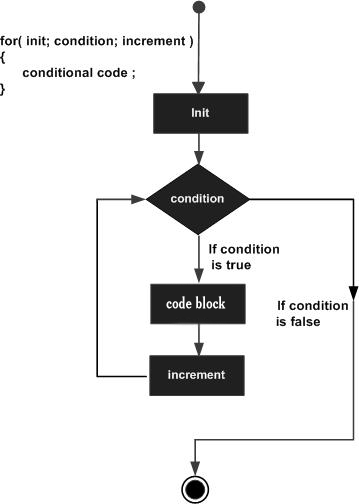# TypeScript 循环## for 循环

TypeScript for 循环用于多次执行一个语句序列，简化管理循环变量的代码。

### 语法

```for ( init; condition; increment ){
statement(s);
}
```

1. init 会首先被执行，且只会执行一次。这一步允许您声明并初始化任何循环控制变量。您也可以不在这里写任何语句，只要有一个分号出现即可。
2. 接下来，会判断 condition。如果为 true，则执行循环主体。如果为 false，则不执行循环主体，且控制流会跳转到紧接着 for 循环的下一条语句。
3. 在执行完 for 循环主体后，控制流会跳回上面的 increment 语句。该语句允许您更新循环控制变量。该语句可以留空，只要在条件后有一个分号出现即可。
4. 条件再次被判断。如果为 true，则执行循环，这个过程会不断重复（循环主体，然后增加步值，再然后重新判断条件）。在条件变为 false 时，for 循环终止。

condition 可以是任意的表达式，当条件为 true 时执行循环，当条件为 false 时，退出循环。

### 流程图## TypeScript

var num:number = 5; var i:number; var factorial = 1; for(i = num;i>=1;i--) { factorial *= i; } console.log(factorial)

## JavaScript

var num = 5; var i; var factorial = 1; for (i = num; i >= 1; i--) { factorial *= i; } console.log(factorial);

```120
```

## for...in 循环

for...in 语句用于一组值的集合或列表进行迭代输出。

### 语法

```for (var val in list) {
//语句
}
```
val 需要为 string 或 any 类型。

## TypeScript

var j:any; var n:any = "a b c" for(j in n) { console.log(n[j]) }

## JavaScript

var j; var n = "a b c"; for (j in n) { console.log(n[j]); }

```a

b

c
```

## for…of 、forEach、every 和 some 循环

for...of 语句创建一个循环来迭代可迭代的对象。在 ES6 中引入的 for...of 循环，以替代 for...in 和 forEach() ，并支持新的迭代协议。for...of 允许你遍历 Arrays（数组）, Strings（字符串）, Maps（映射）, Sets（集合）等可迭代的数据结构等。

## TypeScript for...of 循环

let someArray = [1, "string", false]; for (let entry of someArray) { console.log(entry); // 1, "string", false }

forEach、every 和 some 是 JavaScript 的循环语法，TypeScript 作为 JavaScript 的语法超集，当然默认也是支持的。

## TypeScript forEach 循环

let list = [4, 5, 6]; list.forEach((val, idx, array) => { // val: 当前值 // idx：当前index // array: Array });

## TypeScript every 循环

let list = [4, 5, 6]; list.every((val, idx, array) => { // val: 当前值 // idx：当前index // array: Array return true; // Continues // Return false will quit the iteration });

## while 循环

while 语句在给定条件为 true 时，重复执行语句或语句组。循环主体执行之前会先测试条件。

### 语法

```while(condition)
{
statement(s);
}
```

condition 可以是任意的表达式，当条件为 true 时执行循环。 当条件为 false 时，程序流将退出循环。

### 流程图## TypeScript

var num:number = 5; var factorial:number = 1; while(num >=1) { factorial = factorial * num; num--; } console.log("5 的阶乘为："+factorial);

## JavaScript

var num = 5; var factorial = 1; while (num >= 1) { factorial = factorial * num; num--; } console.log("5 的阶乘为：" + factorial);

```5 的阶乘为：120
```

## do...while 循环

### 语法

```do
{
statement(s);
}while( condition );
```

### 流程图## TypeScript

var n:number = 10; do { console.log(n); n--; } while(n>=0);

## JavaScript

var num = 5; var n = 10; do { console.log(n); n--; } while (n >= 0);

```10
9
8
7
6
5
4
3
2
1
0
```

## break 语句

break 语句有以下两种用法：

1. break 语句出现在一个循环内时，循环会立即终止，且程序流将继续执行紧接着循环的下一条语句。
2. 它可用于终止 switch 语句中的一个 case。

### 语法

```break;
```

### 流程图## TypeScript

var i:number = 1 while(i<=10) { if (i % 5 == 0) { console.log ("在 1~10 之间第一个被 5 整除的数为 : "+i) break // 找到一个后退出循环 } i++ } // 输出 5 然后程序执行结束

## JavaScript

var i = 1; while (i <= 10) { if (i % 5 == 0) { console.log("在 1~10 之间第一个被 5 整除的数为 : " + i); break; // 找到一个后退出循环 } i++; } // 输出 5 然后程序执行结束

```在 1~10 之间第一个被 5 整除的数为 : 5
```

## continue 语句

continue 语句有点像 break 语句。但它不是强制终止，continue 会跳过当前循环中的代码，强迫开始下一次循环。

### 语法

```continue;
```

### 流程图## TypeScript

var num:number = 0 var count:number = 0; for(num=0;num<=20;num++) { if (num % 2==0) { continue } count++ } console.log ("0 ~20 之间的奇数个数为: "+count) //输出10个偶数

## JavaScript

var num = 0; var count = 0; for (num = 0; num <= 20; num++) { if (num % 2 == 0) { continue; } count++; } console.log("0 ~20 之间的奇数个数为: " + count); //输出 10

```0 ~20 之间的奇数个数为: 10
```

## 无限循环

for 创建无限循环语法格式：

```for(;;) {
// 语句
}```

```for(;;) {
console.log("这段代码会不停的执行")
}```

while 创建无限循环语法格式：

```while(true) {
// 语句
} ```

```while(true) {
console.log("这段代码会不停的执行")
}```